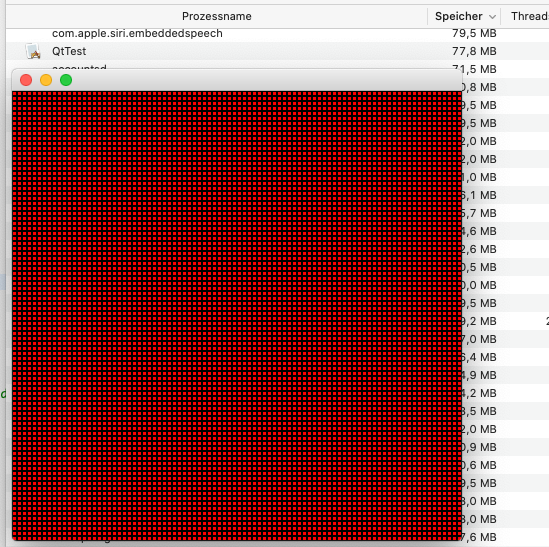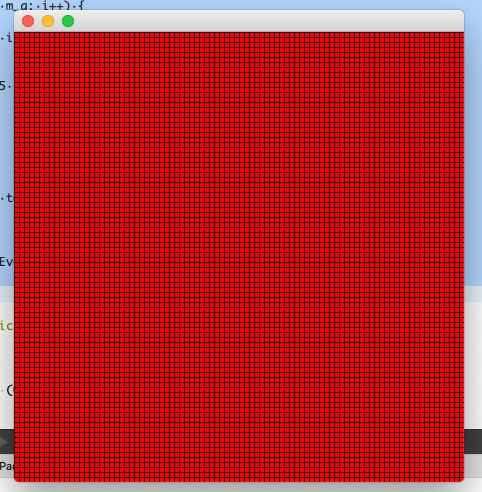# Is there a maximum number of QLabels in a screen?

• Hello,
My code:

``````    int a=90;
label.resize(a);

for(int i=0;i<a;i++)
label[i].resize(a);

for(int i=0;i<a;i++)
{
for(int j=0;j<a;j++)
{
label[i][j]=new QLabel(this);

label[i][j]->setGeometry(i*5,j*5,5,5);
label[i][j]->setStyleSheet("QLabel {background:red; border: 1px solid black}");
}
}
``````

( label is QVector with QLabels ).

When I set a=80 everything is ok. When I change it to 81 my app is crashed.

When I use QGridLayout and add to it QLabels result is the same - 80 ( ok ), 81 (not ok ).

So maximum number of QLabels is between 6400 ( 80^2) and 6561 (81^2)?

EDIT I think my pc can display many QLabels :)

• @TomNow99

Works perfectly fine for me.

``````int main (int argc, char *argv[])
{

QApplication app(argc, argv);

QVector<QVector<QLabel*> > label;

int a=90;
label.resize(a);

for(int i=0;i<a;i++)
label[i].resize(a);

for(int i=0;i<a;i++)
{
for(int j=0;j<a;j++)
{
label[i][j]=new QLabel(nullptr);

label[i][j]->setGeometry(i*5,j*5,5,5);
label[i][j]->setStyleSheet("QLabel {background:red; border: 1px solid black}");
}
}
return 0;
}
``````

• @J-Hilk When I run your code it works, but doesn't see any QLabels. You don't set parent.

• @TomNow99

``````int main (int argc, char *argv[])
{

QApplication app(argc, argv);

QVector<QVector<QLabel*> > label;

int a=90;
label.resize(a);

for(int i=0;i<a;i++)
label[i].resize(a);

QWidget w;
w.resize(a * 5, a* 5);

for(int i=0;i<a;i++)
{
qDebug() << i << "of" << a;
for(int j=0;j<a;j++)
{
label[i][j]=new QLabel(&w);

label[i][j]->setGeometry(i*5,j*5,5,5);
label[i][j]->setStyleSheet(QStringLiteral("QLabel {background:red; border: 1px solid black}"));
}
}

w.show();

qDebug() << "Now showing";

return app.exec();
}
``````

• @J-Hilk Still crashed. So this is my pc problem. Thank you for help :)

• @TomNow99
I ran it in release, because in debug it took quite literally forever to create that many QLabels

only 70mb ram, is consumed so I doubt thats the problem:that said, if your only objective is to create such a grid, you can do that easily with overwriting paint event on a QWidget class:

``````class SomeClass : public QWidget
{
Q_OBJECT

public:
explicit SomeClass(int a, QWidget *parent = nullptr)
: QWidget(parent), m_a(a)
{
resize(5 * a, 5 * a);
}

protected:
int m_a;
virtual void paintEvent(QPaintEvent *event) override{
QPainter p(this);

p.fillRect(rect(), Qt::red);
p.setBrush(QBrush(Qt::black));
for(int i (0); i < m_a; i++) {
//Vertical
p.drawLine(5 * i, 0, 5 * i, height());

//Horizontal
p.drawLine(0, 5 * i, width(), 5 * i);
}
}
};
``````
``````int main (int argc, char *argv[])
{

QApplication app(argc, argv);

SomeClass s(90);
s.show();

return app.exec();
}
``````• @TomNow99
Maximum number of widgets should only be limited by memory. However, performance & resources will be hit. You simply should not create 6.5K labels, whether it works or not. Next you'll be wanting to do a `connect()` on each one, with 6.5K signal/slots :) @J-Hilk has shown a way of creating a grid just via painting, you should be looking for a solution like that or similar.

This, by the way, is also the reason why one-man crusaders like @VRonin are on a mission to rid the world of `QTableWidget::setCellWidget()` --- can create too many individual widgets when used in a table, where a `QStyledItemDelegate` can do the job via drawing instead of creating actual widgets.